# RD Sharma Class 8 Solutions Chapter 10 Direct and Inverse variations Ex 10.1

In this chapter, we provide RD Sharma Class 8 Solutions Chapter 10 Direct and Inverse variations Ex 10.1 for English medium students, Which will very helpful for every student in their exams. Students can download the latest RD Sharma Class 8 Solutions Chapter 10 Direct and Inverse variations Ex 10.1 Maths pdf, free RD Sharma Class 8 Solutions Chapter 10 Direct and Inverse variations Ex 10.1 book pdf download. Now you will get step by step solution to each question.

### RD Sharma Solutions for Class 8 Chapter 10 Direct and Inverse variations Ex 10.1Download PDF

Question 1.
Explain the concept of direct variation.
Solution:
If two quantifies a and b vary with each other in such a way that the ratio ab remains constant and is positive, then we say that a and b vary directly with each other or a and b are in direct variation.

Question 2.
Which of the following quantities vary directly with each other ?
(i) Number of articles (x) and their price (y).
(ii) Weight of articles (x) and their cost (y).
(iii) Distance x and time y, speed remaining the same.
(iv) Wages (y) and number of hours (x) of work.
(v) Speed (x) and time (y) (distance covered remaining the same).
(vi) Area of a land (x) and its cost (y).
Solution:
(i) It is direct variation because more articles more price and less articles, less price.
(ii) It is direct variation because, more weight more price, less weight, less price.
(iii) It is not direct variation. The distance and time vqry indirectly or inversely.
(iv) It is direct variation as more hours, more wages, less hours, less wages.
(v) It is not direct variation, as more speed, less time, less speed, more time.
(vi) It is direct variation, as more area more cost, less area, less cost.
Hence (i), (ii), (iv) and (vi) are in direct variation.

Question 3.
In which of the following tables x and y vary directly ?Solution: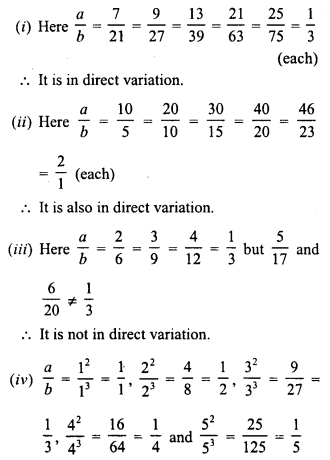All are different.
It is not in direct variation.
Hence (i) and (ii) are in direct variation.

Question 4.
Fill in the blanks in each of the following so as to make the statement true :
(i) Two quantities are said to vary ……….. with each other if they increase (decrease) together in such a way that the ratio of the corresponding values remains same.
(ii) x and y are said to vary directly with each other if for some positive number k = k.
(iii) If u = 3v, then u and v vary ……….. with each other.
Solution:
(i) Two quantities are said to vary directly with each other if they increase (decrease) together in such a way that the ratio of the corresponding values remains same.
(ii) x and y are said to vary directly with each other if for some positive number k, xy = k.
(iii) If u = 3v, then u and v vary directly with each other.

Question 5.
Complete the following tables given that x varies directly as y.Solution: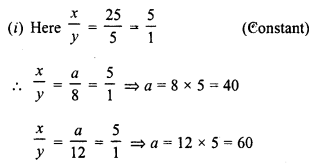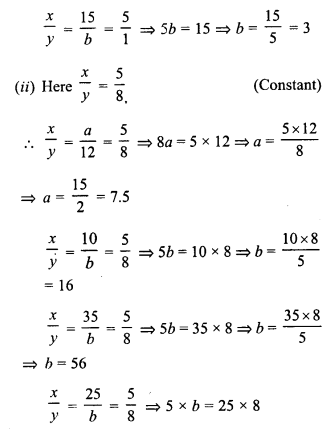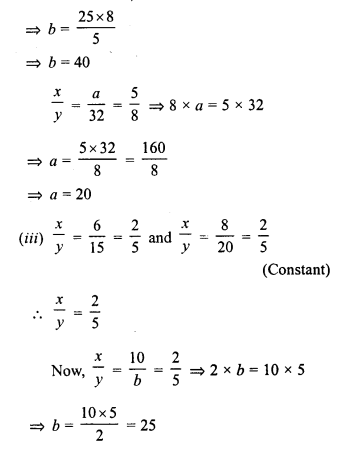9

Question 6.
Find the constant of variation from the table given below :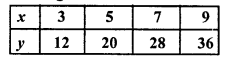Solution:Set up a table and solve the following problems. Use unitary method to verify the answer.
Question 7.
Rohit bought 12 registers for Rs. 156, find the cost of 7 such registers.
Solution:
Price of 12 registers = Rs. 156
Let cost of 7 registers = Rs. x. ThereforeQuestion 8.
Anupama takes 125 minutes in walking a distance of 100 metre. What distance would she cover in 315 minutes.
Solution:
For walking 100 m, time is taken = 125 minutes
Let in 315 minutes, distance covered = m
Therefore,Question 9.
If the cost of 93 m of a certain kind of plastic sheet is Rs. 1395, then what would it cost to buy 105 m of such plastic sheet.
Solution:
Cost of 93 m of plastic sheet = Rs. 1395
Let cost of 105 m of such sheet = Rs. x
Therefore,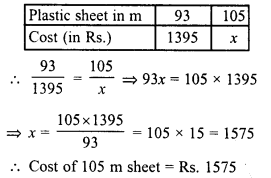Question 10.
Suneeta types 1080 words in one hour. What is GWAM (gross words a minute rate) ?
Solution:
1080 words were typed in = 1 hour = 60 minutes
Let x words will be typed in 1 minute
Therefore,Question 11.
A car is travelling at the average speed of 50 km/hr. How much distance would it travel in 12 minutes.
Solution:
Speed of car = 50 km/hr = 50 km in 60 minutes
Let it travel x km in 12 minutes. ThereforeQuestion 12.
68 boxes of a certain commodity require a shelf length of 13.6 m. How many boxes of the same commodity would occupy a shelf of 20.4 m ?
Solution:
For 68 boxes of certain commodity is required a shelf length of 13.6 m
Let x boxes are require for 20.4 m shelf ThenQuestion 13.
In a library 136 copies of a certain book require a shelf length of 3.4 metre. How many copies of the same book would occupy a shelf-length of 5.1 metres ?
Solution:
For 136 copies of books require a shelf of length = 3.4 m
For 5.1 m shelf, let books be required = x Therefore :Question 14.
The second class railway fare for 240 km of journey is Rs. 15.00. What would be the fare for a journey of 139.2 km ?
Solution:
Fare of second class for 240 km = Rs. 15.00
Let fare for 139.2 km journey = Rs. x
Therefore :Question 15.
If the thickness of a pile of 12 cardboards is 35 mm, find the thickness of a pile of 294 cardboards.
Solution:
Thickness of a pile of 12 cardboards = 35 mm.
Let the thickness of a pile of 294 cardboards = x mm
Therefore :Question 16.
The cost of 97 metre of cloth is Rs. 242.50. What length of this can be purchased for Rs. 302.50 ?
Solution:
Cost of 97 m of cloth = Rs. 242.50
Let x m can be purchase for Rs. 302.50
Therefore :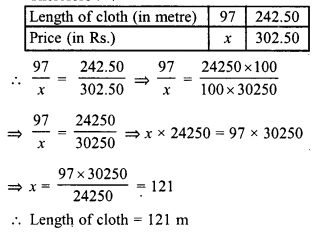Question 17.
men can dig 634 metre long trench in one day. How many men should be employed for digging 27 metre long trench of the same type in one day ?
Solution:
11 men can dig a trench = 634 m long
Let x men will dig a trench 27 m long.
Therefore,Question 18.
A worker is paid Rs. 210 for 6 days work. If his total income of the month is Rs. 875, for how many days did he work ?
Solution:
Payment for 6 day’s work = Rs. 210
Let payment for x day’s work = Rs. 875
Therefore :Question 19.
A worker is paid Rs. 200 for 8 days work. If he works for 20 days, how much will he get ?
Solution:
Labour for 8 days work = Rs. 200
Let x be the labour for 20 days work, thenQuestion 20.
The amount of extension in an elastic string varies directly as the weight hung on it. If a weight of 150 gm produces an extension of 2.9 cm, then what weight would produce an extension of 17.4 cm ?
Solution:
150 gm of weight produces an extension = 2.9 cm
Let x gm of weight will produce an extension of 17.4 cm
Therefore :Question 21.
The amount of extension in an elastic spring varies directly with the weight hung on it. If a weight of 250 gm produces an extension of 3.5 cm, find the extension produced by the weight of 700 gm.
Solution:
A weight of 250 gm produces an extension of 3.5 cm.
Let a weight of 700 gm will produce an extension of x cm. Therefore :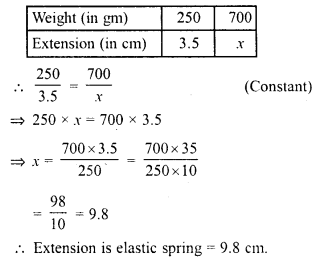Question 22.
In 10 days, the earth picks up 2.6 x 108 pounds of dust from the atmosphere. How much dust will it pick up in 45 days.
Solution:
In 10 days dust is picked up = 2.6 x 108 pounds
Let x pounds of dust is picked up in = 45 days
Therefore,Question 23.
In 15 days, the earth picks up 1.2 x 108 kg of dust from the atmosphere. In how many days it will pick up 4.8 x 10s kg of dust ?
Solution:
Dust of 1.2 x 108 kg is picked up in = 15 days
Let the dust of 4.8 x 108 will be picked up in x days
Therefore,All Chapter RD Sharma Solutions For Class 8 Maths

—————————————————————————–

All Subject NCERT Exemplar Problems Solutions For Class 8

All Subject NCERT Solutions For Class 8

*************************************************

I think you got complete solutions for this chapter. If You have any queries regarding this chapter, please comment on the below section our subject teacher will answer you. We tried our best to give complete solutions so you got good marks in your exam.

If these solutions have helped you, you can also share rdsharmasolutions.in to your friends.